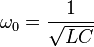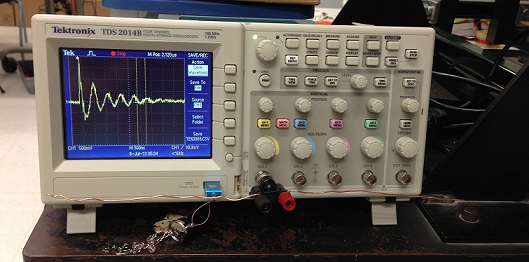# Frequency analysis

### Wave created by LC circuit

As we know, the basic physic model of oscillatory discharge is an LC circuit, whose resonance frequency is given by.  Therefore by varying the length of wire loop used for discharge, we will be able to get waves of different frequency. Here we use single wire loops with length of 1m, 2m, 3m and 4m to discharge the same Leyden jar (the parameter of this Leyden jar can be found here).

The inductance of the wire loop can be calculated by, or online calculator.

Following is the calculated results and measured results. The resonance frequency is then calculated using C=1800pF:

 Loop size: Calculated value(H) Freq=1/(2*PI*SQRT(LC)) (Hz) 1m, R~=50cm, d=1mm 3.95467E-06 1886378.609 2m, R~=60cm, d=1mm 4.88307E-06 1697608.166 2m, R~=90cm, d=1mm 7.78317E-06 1344638.442 3m, R~=120cm, d=1mm 1.08114E-05 1140888.843

The setup is shown below:### Wave detection

The wave is detected using an oscilloscope with two short wires attached which function as an antenna:After the wave was captured by the oscilloscope, it was then saved to a USB and more analysis could be done using computer.

### Results

Below is a summary of the results. According to the equation for LC circuit, the theoretical  ratio between two frequency equals to the square root of the ration of wire length. Click the links to view the waveforms and frequency analysis generated by different wire loops.

 length Frequency(Hz) Avg. Freq.(Hz) Ratio Theoretical ratio 1 1996805 waveform frequency 1m / 2m: 1.240487734 1 2158556 waveform frequency 2077680.5 2m / 4m: 1.319149986 1:2==1.414213562 2 1597444  waveform frequency 2m / 3m: 1.157499627 2:3==1.224744871 2 1752336 waveform  frequency 1674890 3m / 4m: 1.139654783 3:4==1.154700538 3 1484561 waveform  frequency 3 1400000 waveform frequency 3 1456408 waveform frequency 1446989.667 4 1366950 waveform  frequency 4 1214575 waveform frequency 4 1227496 waveform  frequency 1269673.667